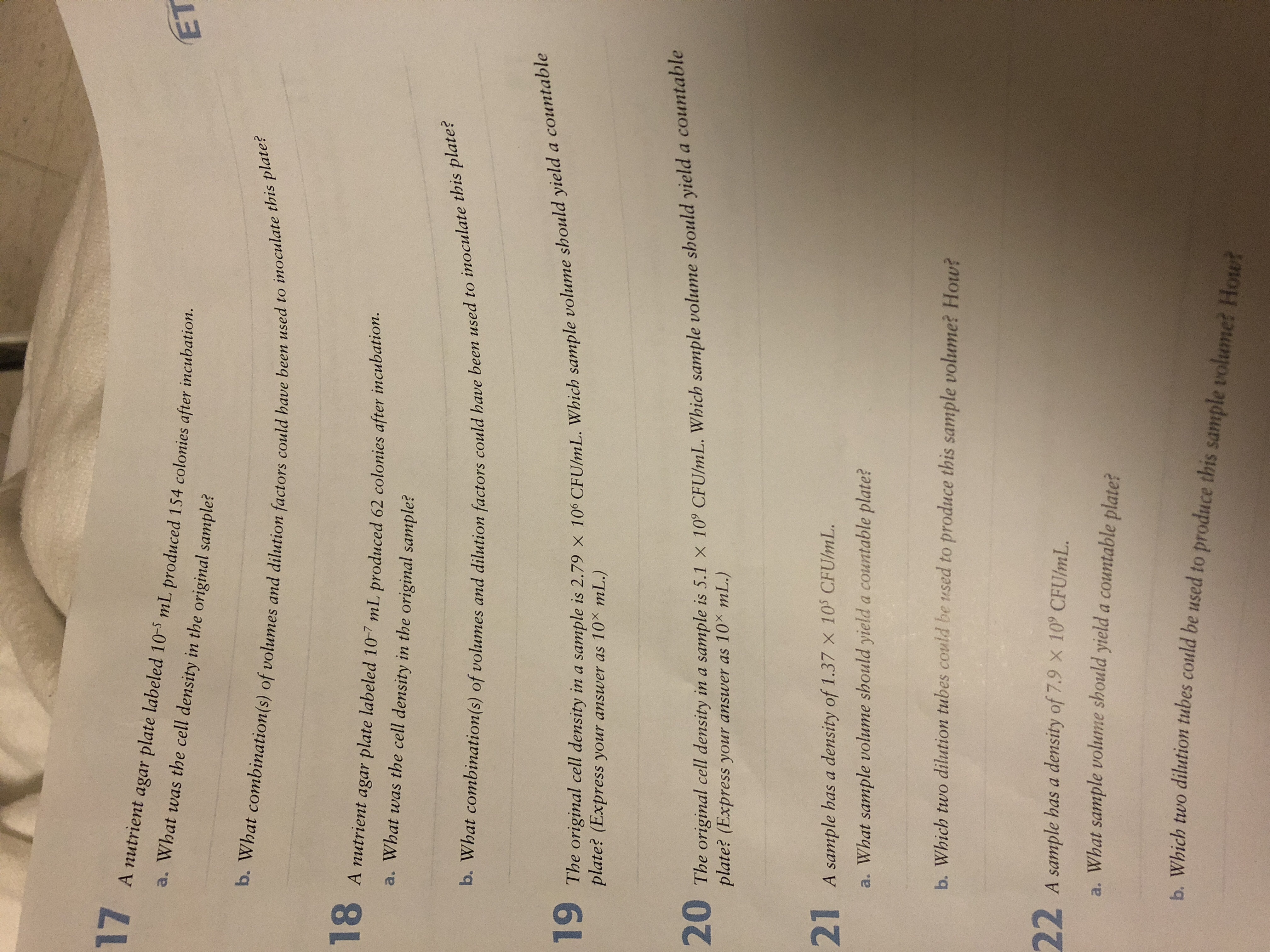# 17A nutrient agar plate labeled 10-5 mL produced 154 colonies after incubation.a. What was the cell density in the original sample?ETb. What combination(s) of volumes and dilution factors could have been used to inoculate this plate?A nutrient agar plate labeled 10-7 mL produced 62 colonies after incubation.a. What was the cell density in the original sample?b. What combination(s) of volumes and dilution factors could have been used to inoculate this plate?The original cell density in a sample is 2.79 x 100 CFU/mL. Which sample volume should yielda countablemL.)plate? (Express your answer as 1020 The original cell density in a sample is 5.1 x 10° CFU/mL. Which sample volume should yield a countableplate? (Express your answer as 10 mL.)21A sample has a density of 1.37 x 105 CFU/mL.a. What sample volume should yield a countable plate?b. Which two dilution tubes could be used to produce this sample volume? How?22A sample has a density of 7.9 x 10° CFUlmL.a. What sample volume should yield a countable plate?h Which two dilution tubes could be used to produce this sample volume? How?

Questionhelp_outlineImage Transcriptionclose17 A nutrient agar plate labeled 10-5 mL produced 154 colonies after incubation. a. What was the cell density in the original sample? ET b. What combination(s) of volumes and dilution factors could have been used to inoculate this plate? A nutrient agar plate labeled 10-7 mL produced 62 colonies after incubation. a. What was the cell density in the original sample? b. What combination(s) of volumes and dilution factors could have been used to inoculate this plate? The original cell density in a sample is 2.79 x 100 CFU/mL. Which sample volume should yielda countable mL.) plate? (Express your answer as 10 20 The original cell density in a sample is 5.1 x 10° CFU/mL. Which sample volume should yield a countable plate? (Express your answer as 10 mL.) 21 A sample has a density of 1.37 x 105 CFU/mL. a. What sample volume should yield a countable plate? b. Which two dilution tubes could be used to produce this sample volume? How? 22 A sample has a density of 7.9 x 10° CFUlmL. a. What sample volume should yield a countable plate? h Which two dilution tubes could be used to produce this sample volume? How? fullscreen
check_circleExpert Solution
Step 1

A dilution:

It can be defined as a method of addition of more solvent to a solution of a definite concentration, thus, to drop the concentration.

If more and more solvent is added to that solution, then the volume of the whole solution increases. While the concentration of the solution declines.

Dilution factor:

It is defined as the ratio of the volume of the original solution (concentrated) to the volume of the absolute solution (dilute).

Step 2
1. The cell density in the original sample were:

As 154 independent colonies were seen on 10-5 mL nutrient agar plate.

It means that there were 154 bacterial cells present, if the original stock culture was diluted to 105 times.

Therefore, in the original stock solution or sample the bacterial cell concentration (or cell density) would be 154 X 105 cells/mL.

Step 3
1. The groupings of volumes and dilution factors that could have been used to inoculate this nutrient plate:

Since the nutrient plate was diluted to 10-5 ml. Then the following volumes and dilution factors could be done.

a). First, 1 ml of the original sample was taken with 999 ml of the diluent. This produced 1:1000 dilution of the sample. Then the concentration of the original solution would be 10-3 ml.

b). Secondly, 1 ml was taken from this sample of 10-3 mL and added to 999 mL of the diluent. Then the solution was dilu...

### Want to see the full answer?

See Solution

#### Want to see this answer and more?

Solutions are written by subject experts who are available 24/7. Questions are typically answered within 1 hour*

See Solution
*Response times may vary by subject and question
Tagged in

### Microbiology